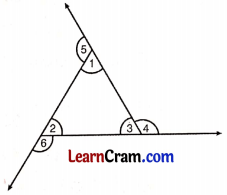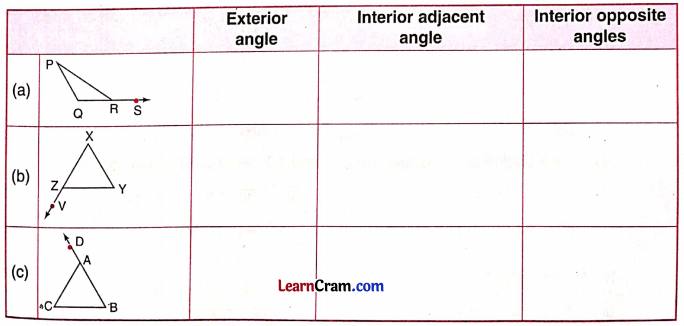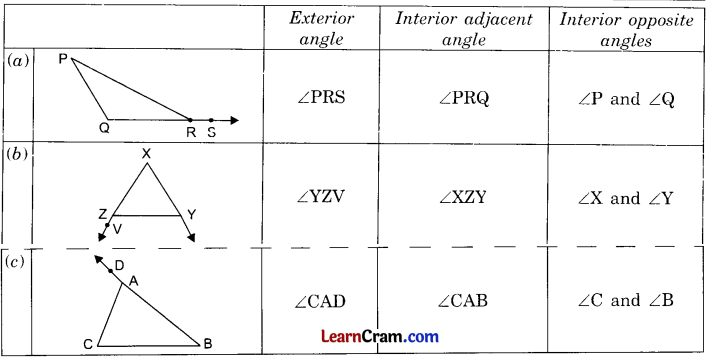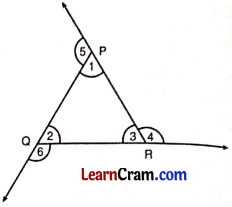# DAV Class 6 Maths Chapter 12 Worksheet 3 Solutions

The DAV Maths Book Class 6 Solutions and DAV Class 6 Maths Chapter 12 Worksheet 3 Solutions of Triangles offer comprehensive answers to textbook questions.

## DAV Class 6 Maths Ch 12 WS 3 Solutions

Question 1.
Name all the exterior angles in the given triangle:∠4, ∠5 and ∠6 are the exterior angles of the given ΔABC.

Question 2.
Complete the table given below:Question 3.
Look at the figure and complete the given statements:(а) Interior opposite angles corresponding to the exterior angle ∠5 are ___________ and ___________.
∠2 and ∠3

(b) Interior adjacent angle corresponding to ∠6 is ___________.